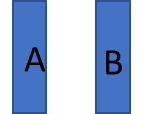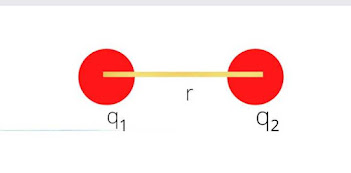## Labels

### ELECTRIC DIPOLE NOTES: ELECTRIC DIPOLE , DIPOLE LENGTH, ELECTRIC FIELD DUE TO ELECTRIC DIPOLE

Electric Dipole: It is a  system of equal and opposite charges separated by some  fixed distance.

DIPOLE LENGTH: the distance AB = 2a is called dipole length. It is a vector quantity , whose direction is from -q to +q.

Dipole Moment (𝑷⃗  ): It is the measure of strength of electric field produced by a dipole It is defined as the product of magnitude of either charge and separation between the charges.

i.e. 𝐏⃗ = q × 𝟐𝒂⃗  By convention, direction of electric dipole moment is always taken from negative to positive charge.

Units of 𝑷⃗  : units of 𝑷⃗  = units of charge × units of distance = Cm

Dimensions of  𝐏⃗  : [𝑷⃗ ] = [q] × [2a] = AT × L = LTA

### ELECTRIC FIELD NOTES : ELECTRIC FIELD

Electric Field:

Electric field is a region of space around a charge or a system of charges within which other charged particles experience electrostatic forces.

Electric Field Strength or Electric Field Intensity or Electric Field:

Electric field strength at a point in an electric field is the electrostatic force per unit positive charge acting on a vanishingly small positive test charge placed at that point.

𝑬 = 𝑭/𝒒₀

The test charge is considered to be vanishingly small  because its presence should not alter the configuration of the source charge and thus the electric field which is intended to be measured.

Since 𝒒₀ is taken positive, the direction of electric field ( E ) is along the direction of electrostatic force ( F ).

Electric field is a vector quantity whose magnitude and direction are uniquely determined at every point in the field.

SI unit of electric field is newton / coulomb ( NC⁻¹ ).

### The electric field produced by a point charge q

The magnitude of the force exerted by the charge q on a test charge q₀ is,

F= \frac{1}{4 πε_{0}} \frac{q_{}q_{0}}{r^{2}}

E= F/q0 =

F= \frac{1}{4 πε_{0}} \frac{q_{}}{r^{2}}

If q is positive, E is directed away from q. On the other hand, if q is negative, then E is directed towards q.

ELECTRIC FIELD INTENSITY DUE TO A GROUP OF CHARGES OR SUPERPOSITION PRINCIPLE :

Electric field intensity at any point due to a group of point charges is equal to the vector sum of electric field intensities due to individual charges at the same point.

Electric lines of force:

The paths, along which the unit positive charge will move due to electrostatic force in the field are called electric lines of force.

The tangent at any point on the electric lines of force gives the direction of electric field at that point.

The properties of electric lines of force are:

• i) Electric lines of force start from positive charge and terminate at negative charge.
• ii) Electric Lines of force never intersect each other ( see explanation below)
• iii) Electric lines of force emerge or terminate at right angle to the surface of charge.
• iv) ..

### COULOMB'S LAW : IMPORTANT QUESTIONS

Q1  Given two point charges q₁ and q₂ such that q₁q₂ < 0. What is the nature of force between them?

Ans. it is possible if  one charge  is  positive and other charge is  negative  in nature. Hence, the force between the two charges will be attractive.

Q2 Given two point charges q₁ and q₂ such that q₁q₂ > 0.what is the nature of force between the two charges?

Ans. As  q₁q₂ > 0  it implies that both the charges are positive in nature. Hence, the force between the two charges will be repulsive.

Q.What is the basic cause of quantisation of charge?

Ans. During the charging process, only integral number of electrons is transferred from one body to the other. Therefore, charge possessed by a body is always an integral multiple of 1.6 x 10⁻¹⁹ C.

Q. How many electrons are present in one coulomb of charge?
Ans: as we know q =ne
n =q/e
n= 1/1.6 x 10⁻¹⁹ C. =  6.25 x 10¹⁸

### PHYSICS NOTES: COULOMB'S LAW PART-1

Electrostatics: - It is that branch of physics that deals with the charges at rest. ‘Electro’ means study of charges and ‘Static’ means at rest.

What is charge? Give types of charges?

Charge: - It is scalar quantity possessed by body by virtue of which the body will show attraction or repulsion on the similar charged bodies.

Types of Charges: - There are two types of Charges Positive charges and Negative charges. Like charges repel each other and unlike attract each other.

What is the cause of charging?

The cause of charging a body is the transfer of electrons from one body to another.

The body which losses electron, becomes positively charged. The body which gains electrons becomes negatively charged.

What is the effect on mass due to charging of a body?

As charging is due to the transfer of electrons. Therefore body which gains electrons becomes negatively charged and its mass increases.

Whereas the body which losses electron becomes positively charged and its mass decreases. This change in mass is negligible as mass of electrons is very small i.e 9.1x 10⁻³¹ kg

What is charging by contact?

Charging by Contact:  When a charged object is touches another object, the other object also gets charged with same polarity due to charge transfer.  This is called charge by contact.

What is charging by Induction?

When a charged object is brought closer to another object (not touched), the original object doesn’t lose any charge and the other object gets charged as well with opposite polarity. The other extreme end of the newly charged object develops polarity same as that of the charged object. This type of charging is called charge by induction.

 Properties of Electric Charge 1. Like charges repel while unlike charges attract each other. The true test of electrification is repulsion and not attraction as attraction may also take place between a charged and an uncharged body and also between two oppositely charged bodies. 2. Charge is a scalar. ( because charge has only magnitude no direction. 3. Charge is transferable. (Explanation:  When a charged body is put in contact with an uncharged body, the uncharged body becomes charged due to transfer of electrons from one body to the other. If the charged body is positive, it will draw some electrons from the uncharged body and if it is negative then it will transfer some of its excess electrons to the uncharged body) 4. Charge is always associated with mass: Charge cannot exist without mass though mass can exist without charge. 5. Charge is Additive. The total charge on a system   can be obtained by algebraically adding all the  charges  present on that system . 𝑞 = 𝑞1 + 𝑞2 + 𝑞3 For a system containing n  charges , the total charge of the system can be written as, 𝑞 = 𝑞1 + 𝑞2 + 𝑞3 + … … + 𝑞𝑛     6. Electric charge is conserved.   According to the principle of conservation of charges, the charges are neither created nor destroyed; they are only transferred from one body to the other.(Explanation: BEFORE RUBBING TOTAL  CHARGE ON BODY A AND B = 0      after rubbing let 5 electrons transfer from body A to B . Then body B gets -5e charge , then Body will get +5e charge             Total charge after rubbing -5e+ +5e =0) 7 Electric Charge is quantized.   According to the principle of quantization of electric charge, all the changes on a body  are integral multiples of elementary charge( e)  𝑞 = 土 𝑛𝑒 Where n is an integer (zero, a positive or a negative number) and e is the basic unit of charge, that is, the charge carried by an electron or a proton. The value of e is 1.6 × 10⁻¹⁹C What is the cause of quantisation? The cause of quantisation is that charging of body is always due to  the transfer of electron in integral number. ( means electrons never transfer in fraction or decimal form.) Difference between mass and charge Charge :Charge is invariant. It is independent of velocity. Charge causes electromagnetic force. Charge is quantised. Q = ne; where n is an integer and e is the charge of one electron. Charge cannot exist without mass. Charge can either be positive or negative.  Mass: Mass is variant, according to Einstein's equation, when a body is travelling with speed comparable to that of light, its mass changes. Mass causes gravitational force. Mass is not quantised. i.e, mass cannot be expressed as integral multiples of a basic unit. Mass can exist without charge. Mass is always positive. Coulomb's  Law in electrostatic: Coulomb's  Law:  Coulomb's law states that the force of attraction or repulsion between two stationary point changes is directly proportional to the product of the magnitudes of the two charges and inversely proportional to the square of the distance between them.F∝ q_{1}q_{2}  ---(1)F∝ \frac{1}{r^{2}} -- (2)F∝  q_{1}q_{2} \frac{1}{r^{2}}F= K  q_{1}q_{2}\frac{1}{r^{2}}Where k is the constant of proportionality and is called force constant. K = \frac{1}{4 πε_{0}} = 9x10⁹ Nm²C⁻² in SI unit.F= \frac{1}{4 πε_{0}} \frac{q_{1}q_{2}}{r^{2}}{ε_{0}} is called absolute permitivity of free space.  It is equal to 8.854x10⁻¹² farad per meter in free space. Unit of permitivity is N⁻¹m⁻²C²Dimensions of Permittivity: [ M⁻¹ﱣL ⁻³T ⁴A ² ]Permittivity: Permittivity is a measure of how an electric field affects and is affected by a medium.Limitations of Coulomb's law : It is valid only under the following conditions: - 1) The electric charges must be at rest. 2) Electric charges must be point chargesDielectric Constant : The Dielectric constant (K) is defined as  the ratio of the permittivity of the substance to permittivity of the free space ({ε_{0}}). i.e. K = ε/{ε_{0}} . As Permittivity of medium and permittivity of free space both have same units (F/m i.e. Farad/meter) dielectric constant becomes dimensionless quantity. For vacuum or air K = 1 Dielectric constant is also called relative permittivity. Its value is >1 for all media. It is dimensionless quantity. It has no unit.Coulmb's Law in vector form:

As the Colombian forces act along r₁₂ or r₂₁i.e. along the line joining the centers of two charges, so they are central forces.

What is the Principle of Superposition?

The principle of superposition states that every charge in space creates an electric field at point independent of the presence of other charges in that medium. The resultant electric field is a vector sum of the electric field due to individual charges.”

Continuous Charge Distribution: The continuous distribution of charge may be one dimensional , two dimensional or three dimensional.
The ways in which charges can be distributed are :
Linear charge distribution.
Surface charge distribution.
Volume charge distribution

Linear charge distribution:  When the charges get distributed uniformly along a length, like around the circumference of a circle or along a straight wire it is called linear charge distribution.

Linear charge density  is defined as charge distributed per unit length, it is  denoted by  λ .
λ = q/L
and it is unit is Coulombs per meter. ( Cm⁻¹)

(b) Surface charge distribution: When the charges get distributed uniformly over the surface , it is called surface  charge distribution.

Surface charge density  is defined as charge distributed per unit area, it is  denoted by  σ .
σ = q/A  and its unit is Coulombs per meter sqaure. ( Cm⁻²)

Volume charge distribution: When a charge is distributed uniformly over a volume it is said to be volume charge distribution, like distribution of charge inside a sphere, or a cylinder.

Volume  charge density  is defined as charge distributed per unit volume, it is  denoted by  ρ .
ρ = q/V  and its unit is Coulombs per meter  cube. ( Cm⁻³).

### ELECTRIC DIPOLE NOTES: ELECTRIC DIPOLE , DIPOLE LENGTH, ELECTRIC FIELD DUE TO ELECTRIC DIPOLE

Electric Dipole: It is a  system of equal and opposite charges separated by some  fixed distance. DIPOLE LENGTH : the distance AB = 2a is ...